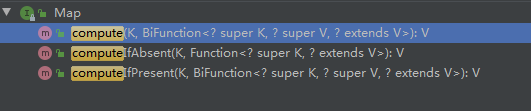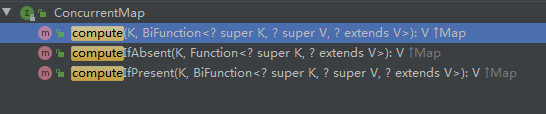## compute简介• compute：计算并更新值
• computeIfAbsent：Value不存在时才计算
• computeIfPresent：Value存在时才计算

## compute有啥用?

/**
* 公众号：Java技术栈
*/
private static void preJava8() {
List<String> animals = Arrays.asList("dog", "cat", "cat", "dog", "fish", "dog");
Map<String, Integer> map = new HashMap<>();
for(String animal : animals){
Integer count = map.get(animal);
map.put(animal, count == null ? 1 : ++count);
}
System.out.println(map);
}


{cat=2, fish=1, dog=3}

Java 8 compute 实现方式：

/**
* 公众号：Java技术栈
*/
private static void inJava8() {
List<String> animals = Arrays.asList("dog", "cat", "cat", "dog", "fish", "dog");
Map<String, Integer> map = new HashMap<>();
for(String animal : animals){
map.compute(animal, (k, v) -> v == null ? 1 : ++v);
}
System.out.println(map);
}


## compute源码分析

/**
* 公众号：Java技术栈
*/
default V compute(K key,
BiFunction<? super K, ? super V, ? extends V> remappingFunction) {

// 函数式接口不能为空
Objects.requireNonNull(remappingFunction);

// 获取旧值
V oldValue = get(key);

// 获取计算的新值
V newValue = remappingFunction.apply(key, oldValue);

if (newValue == null) { // 新值为空
// delete mapping
if (oldValue != null || containsKey(key)) { // 旧值存在时
// 移除该键值
remove(key);
return null;
} else {
// nothing to do. Leave things as they were.
return null;
}
} else { // 新值不为空
// 添加或者覆盖旧值
put(key, newValue);
return newValue;
}
}


https://github.com/javastacks/javastack